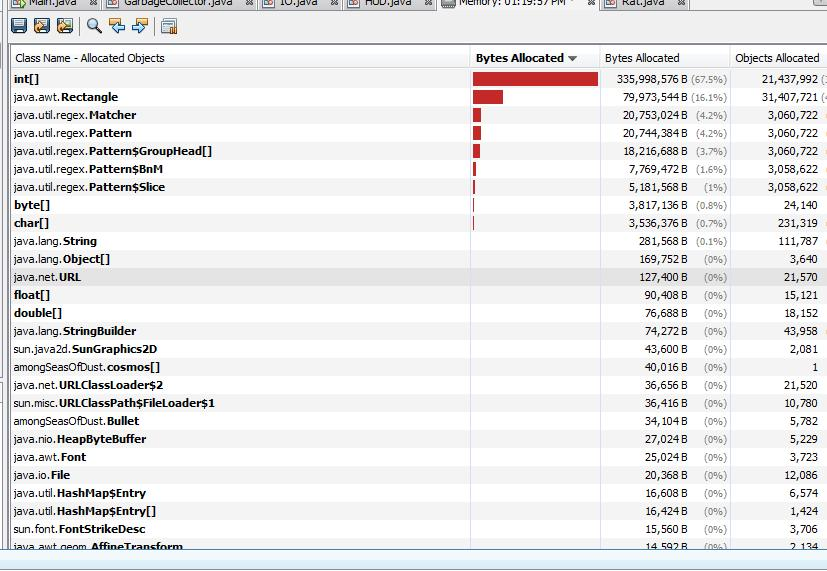Where has all the Memory Gone?

As I have been working on ASoD 0.75, i have wondered where exactly all my RAM is going. So, I started up a profiler and got some information. The purpose of this article is to educate you on where the RAM in ASoD is going and inform you of plans to optimize the system even further.

Posted by on

Introduction
As I have been working on ASoD 0.75, i have wondered where exactly all my RAM is going. So, I started up a profiler and got some information. The purpose of this article is to educate you on where the RAM in ASoD is going and inform you of plans to optimize the system even further.

The Chart
The profiling was less than a minute, I just went from one solar system to another, docked, and exited. The RAM fluctuated by about 20% as the garbage collection did its work. Here is the important part of the output.The immediate conclusion is that the almighty integer has outperformed by far every other type of variable in memory consumption. Indeed, the runner up is a mere 16.1% memory usage to the integer's 67.5% .

Reasons for the Integer to Rank Top
ASoD does not work with doubles or floating points often, and since everything is based on pixels it is all integer form. Integers are used in the many For loops ASoD needs, and are used to define properties such as behaviors. They declare the type of object something is while still finding time to store the sleugh of statistics and numbers needed for player-environment interaction.

``````space = new cosmos(0, 5300, 5100, "Station 1");
space.setPlanetTexture(Toolkit.getDefaultToolkit().getImage(getUrl("station_one.png")));
space.solar = "Marcina";
space.dockable = true;
space.setDockedTexture(Toolkit.getDefaultToolkit().getImage(getUrl("slasher_station_docked.png")));
space.selling = new String[]{"Raw Ore", "Weapons", "Argon"};
space.sellingPrices = new int[]{15, 5, 80};
space.buying = new String[]{"Equipment", "Water", "Food", "Ice", "Oxygen", "Narcotics", "Nitrogen", "Carbon Dioxide", "Pirated Software"};
space.buyingPrices = new int[]{20, 30, 20, 9, 30, 150, 18, 20, 1500};
space.availableShips = new String[]{"Slasher Frigate", "Slasher Miner"};
space.shipCost = new int[]{8200, 9500};
space.faction = "Slasher";
space.dockable = true;``````

In the above code you can see many of the usages of the integer in ASoD. It can be seen defining coordinates, prices, indexes, and object type.

All hail the Rectangle
Without a rectangle there would be no game development at all. Rectangles are perfect shapes for 2D collision testing and other things. The rectangle was the runner up for memory consumption and is the easiest to put on a diet.

Whenever a method runs and creates a local variable, CPU and memory operations are done. At the end of the method the variable is discarded. While for integers this method will be a pain to make all variables global to avoid creation/destruction, the Rectangle is easier. First of all the rectangles created typically need to be shared. In addition some sloppy coding on my part has some global rectangles recreated all-too-often.

``````    public Rectangle getBounds() {
searchForTargetRect = new Rectangle(x - 300, y - 300, 600, 600);
if (this.targetSpace == 50) {
this.targetSpace = -100;
this.targetRat = -100;
}
Rectangle r = null;
try {
//this.cleanUpNonAgressedHoldStillRats();
if (type == -50) {
r = new Rectangle(x, y, 60, 60);
}
if (type == -1) {
r = new Rectangle(x, y, 60, 60);
}
if (type == 0) {
r = new Rectangle(x, y, 50, 50);
}
if (type == 1) {
r = new Rectangle(x + 10, y + 10, 80, 70);
}
if (type == 2) {
r = new Rectangle(x, y, 50, 50);
}
if (type == 3) {
r = new Rectangle(x, y, 200, 200);
}
if (type == 4) {
r = new Rectangle(x, y, 50, 50);
}
if (type == 5) {
r = new Rectangle(x, y, 55, 55);
}
if (type == 6) {
r = new Rectangle(x, y, 120, 120);
}
if (type == 7) {
r = new Rectangle(x, y, 210, 210);
}
if (type == 8) {
r = new Rectangle(x, y, 100, 100);
}
if (type == 9) {
r = new Rectangle(x, y, 140, 140);
}
if (type == 10) {
r = new Rectangle(x, y, 120, 120);
}
} catch (Exception e) {
}
return r;
}``````

The above code is called very often by everything from bullets to NPC navigation. It creates a new bounds every time its called because this is convenient. However, it is likely adding a massive portion of crap variable work that can be avoided. So, by making the rectangle bounds declared at NPC creation and this method only Return the Rectangle, it can save memory operations.

Why Bother?
Seriously, why should I bother? Well, its because in my tests the RAM used by ASoD fluctuated by the second between +20% normal and -20% normal (approximately). This is Java's internal garbage collector picking away these temporary variables and causing lag in the process (often manifesting as a jump or skip in the game every second or so).

ASoD has been optimized and reoptimized, but it still has a lot of potential for slimness. One of the original goals of it was not to waste resources, so I think a round of reoptimization focusing on Variables instead of Procedures is in order.